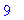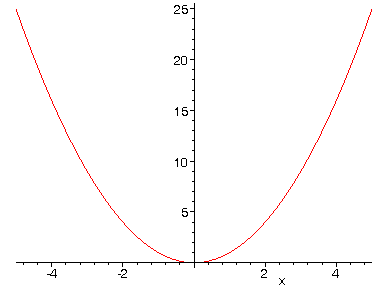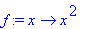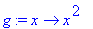FUNCTIONS VS EXPRESSIONS

In MAPLE, you can work with either expressions or functions. Sometimes one format is easier to work with, and sometimes the other. Below we illustrate how to set up, evaluate, and graph each. First we'll look at expressions, then a function, and then illustrate how to turn an expression into a function.

EXPRESSIONS:

> y:=x^2;> subs(x=3, y);> plot(y, x=-5..5);FUNCTIONS:

> f:=x->x^2;> f(3);> plot(f(x), x=-5..5);EXPRESSION TO FUNCTION CONVERSION:

> g:=unapply(x^2, x);> g(3);